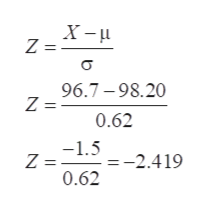Find the z- score corresponding to the given value and use the z- score to determine whether the value is unusual. Consider a score to be unusual if its z- score is less than -2.00 or greater than 2.00.  Round the z- score to the nearest tenth if necessary. A body temperature of 96.7 F given that human body temperatures have a mean of 98.20 F and a standard deviation of 0.62 F.Please include detailed steps

Question

Find the z- score corresponding to the given value and use the z- score to determine whether the value is unusual. Consider a score to be unusual if its z- score is less than -2.00 or greater than 2.00.  Round the z- score to the nearest tenth if necessary.

A body temperature of 96.7 F given that human body temperatures have a mean of 98.20 F and a standard deviation of 0.62 F.

Please include detailed steps

Step 1

Given body temperature 96.7 F
Mean = 98.20 F
Standard deviation = 0.62 F

Z score is the distance of the data point from mean in terms os standard deviatio...help_outlineImage TranscriptioncloseX- Z = 96.7-98.20 Z = 0.62 -1.5-2.419 Z = 0.62 fullscreen

Want to see the full answer?

See Solution

Want to see this answer and more?

Our solutions are written by experts, many with advanced degrees, and available 24/7

See Solution
Tagged in

Statistics